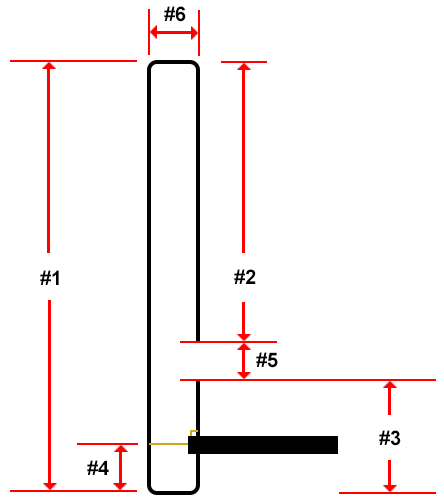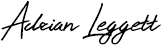## Slim-Jim antenna calculator

I have just finished my Slim Jim antenna calculator and will be using it to build a Slim Jim in my workshop. Photo’s and video coming soon.

Click on the continue button to see more.

Slim Jim antenna calculator

Antenna design

See below the J-Pole antenna design.Calculator explained

Frequency (MHz): Input the frequency for calculations

Velocity factor:  The conductive material user for the antenna’s radiating element will affect how well RF propagates through it and certain materials can slow the RF.

Speed of light is used to determine the speed at which radio waves are travelling in free space. Conductors such as copper, aluminium and steel will slow RF down. Velocity factor (VF)  needs to be taken into account when calculating your antenna.

A common conductor used in antenna construction is copper. Copper slows RF by around 5% so the VF will be 0.95 (95% speed of light).

This calculator uses 0.95 as default but this can be changed depending on what material you will be using to make your radiator.

Inputs

Wavelength (m): 300 / frequency in MHz

Actual wavelength (m): (300 * velocity factor) / frequency in MHz

#1 – Overall length(cm): (22500 * velocity factor) / frequency in MHz

In calculation #2 I have deducted 1/2 the length of the calculated gap (#5)

#2 – 1/2 wave radiator section (cm): (30000 * (0.5 * velocity factor / frequency in MHz)) – ((300/frequency in MHz)/2)

In calculation #3 I have deducted 1/2 the length of the calculated gap (#5)

#3 – 1/4 wave radiator section (cm): (30000 * (0.25 * velocity factory) / frequency in MHz)) – ((300/frequency in MHz)/2)

#4 – 50 ohm feed point (cm): (30000 * (0.025 * velocity factor)) / frequency in MHz

#5 – Gap (cm): 300 / frequency in MHz

#6 – Spacing (cm): (30000 * 0.02175) / frequency in MHz

Note: #2 + #3 + #5 = #1

Total radiator length (cm / m) = (((#1 + #2 + #3) + (#6* 2)) – #5)

Why not take a look at my YouTube channel here or Twitter posts here.

You can also register for my website here.

Thanks

73 de M0NWK